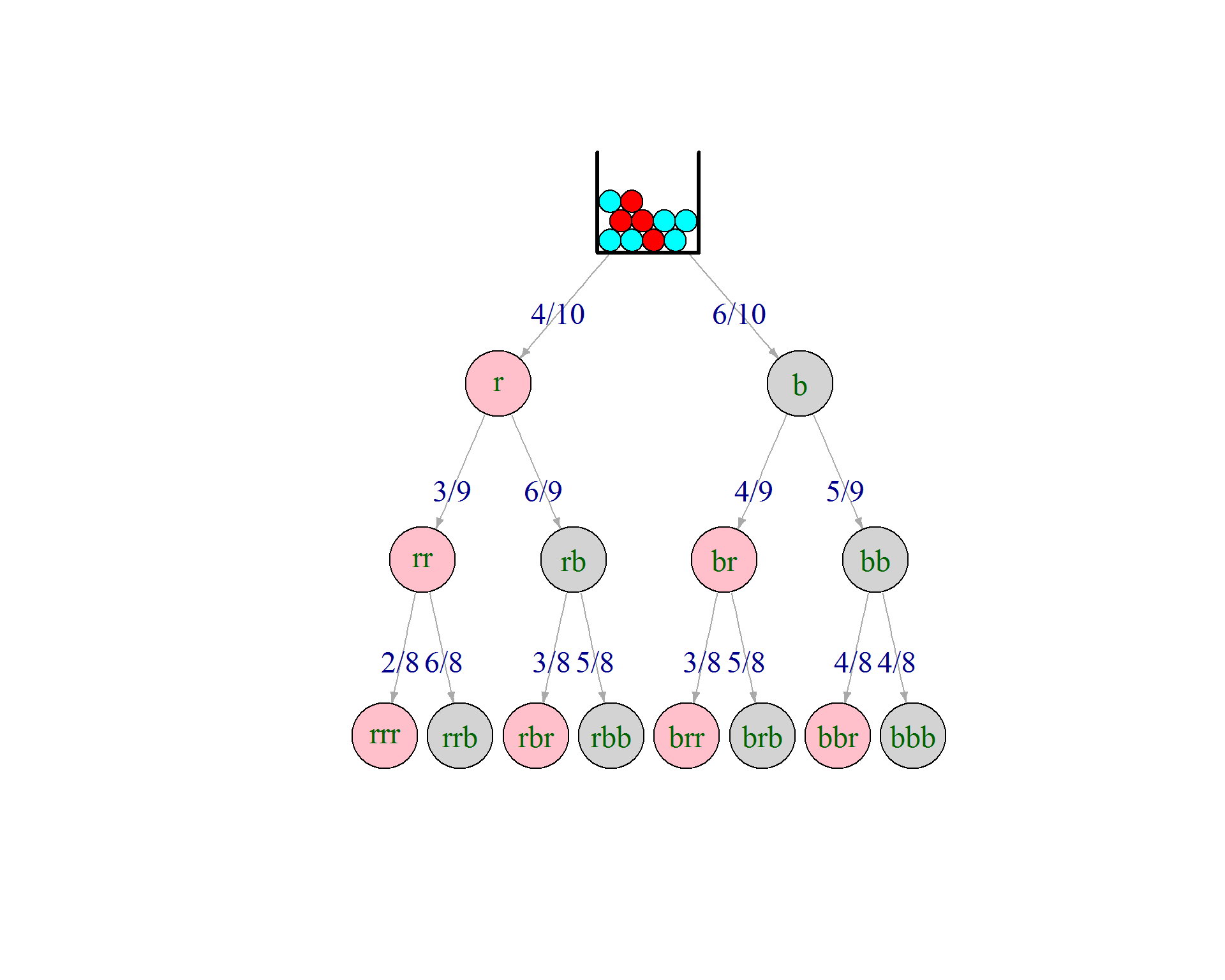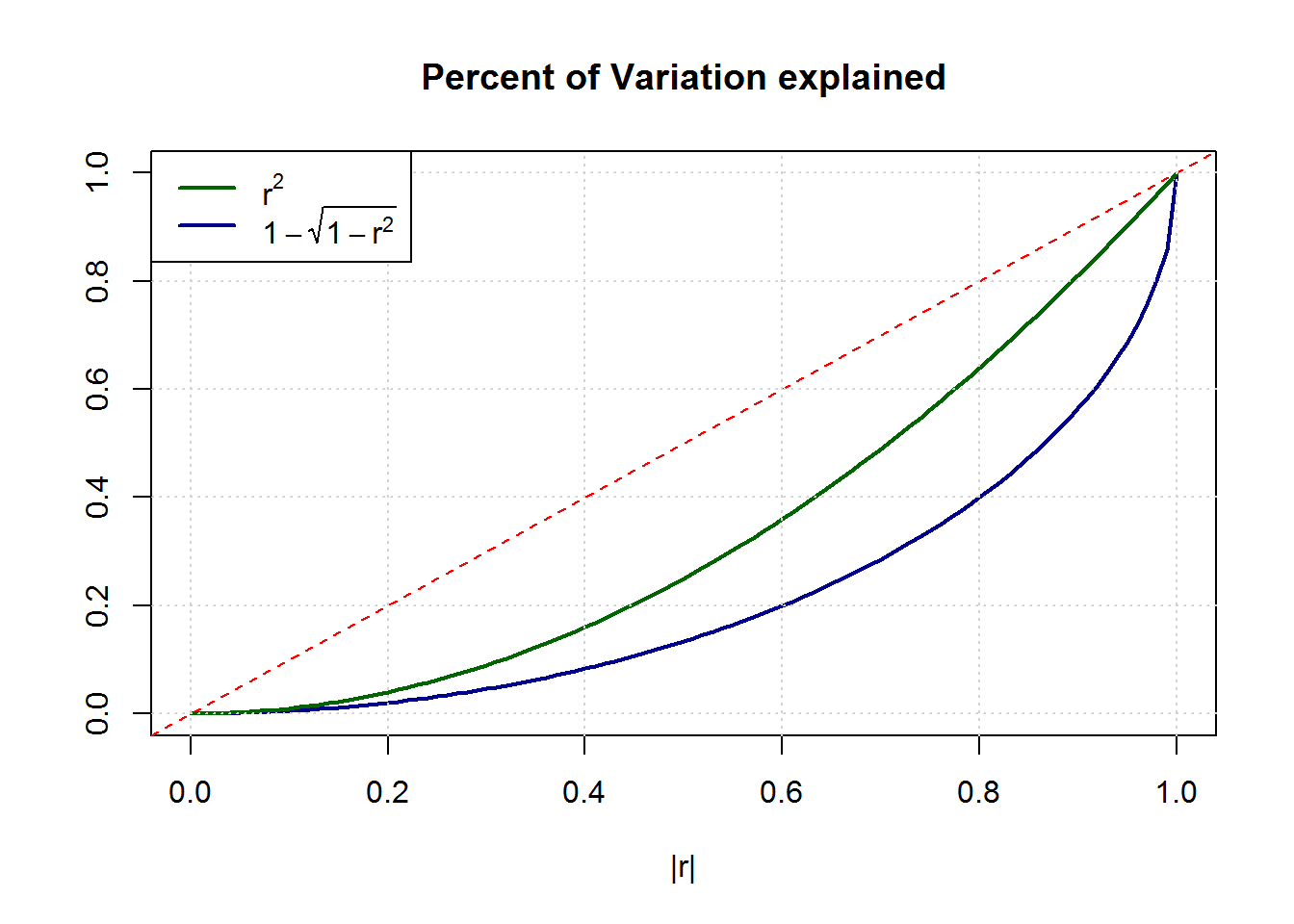## Alternative to the Hypergeometric Formula

The Hypergeometric Distribution is usually explained via an urn analogy and formulated as the ratio of “favorable outcomes” to all possible outcomes:

$\displaystyle \boxed{P(x=a;N,A,n,a) = \frac{{A \choose a} \cdot {N-A \choose n-a} }{{N \choose n}}}$

where $$N$$ is the total number of balls in, $$A$$ the number of “red” balls in the urn, and $$n$$ the sample size. The question answered by the above expression is the probability of finding $$x=a$$ red balls in the sample.

However, a different – equally worthy – viewpoint is that of a tree with conditional probabilities.

Here is an example for $$N=10, A=4, n=3$$So there are 3 leafs with exactly one red ball in the sample. Following the tree we multiply the conditional probabilities of the tree egdes to get to the “and” probability of the leafs. It should be clear that all three probabilites are identical – but for the order of multiplication:

$P (\mbox{one red}) = 3 \cdot P_{leaf} = 3 \cdot \frac{4}{10} \cdot \frac{6}{9} \cdot \frac{5}{8} = 3 \cdot \frac{4 \cdot 6 \cdot 5}{10 \cdot 9 \cdot 8}$

### Alternative formula

How many leafs contain exactly one red ball? Exactly $${n \choose a} = {3 \choose 1} = 3$$. The probability for the precise event “a red balls followed by n-a blue balls”is:

$\frac{A}{N} \cdot \frac{A-1}{N-1} \cdots \frac{A-a+1}{N-a+1} \cdot \frac{N-A}{N-a} \cdot \frac{N-A-1}{N-a-1} \cdots \frac{N-A-(n-a-1)}{N-a-(n-a-1)}$
The last denominator is simply $$N-n+1$$, i.e. the full denominator is $$_NV_n=N!/(N-n)!$$

The left numerator is simply $$_AV_n = A!/(A-a)!$$ in analogy the right numerator $$_{N-A}V_{n-a} = (N-A)!/(N-A-(n-a))!$$ So, all in all ${n \choose a} \cdot \frac{_AV_n \cdot _{N-A}V_{n-a}}{_NV_n} = {n \choose a} \cdot \frac{A! \cdot (N-A)! \cdot (N-n)!}{(A-a)! \cdot (N-A-(n-a))! \cdot N!}$

$= {n \choose a} \cdot \frac{\frac{A!}{(A-a)!} \cdot \frac{(N-A)!}{(N-A-(n-a))!} }{\frac{N!}{(N-n)!}} = \frac{{A \choose a} \cdot {N-A \choose n-a} }{{N \choose n}}$

which leaves us with $\displaystyle \boxed{P(x=a;N,A,n,a) = {n \choose a} \cdot \frac{_AV_n \cdot _{N-A}V_{n-a}}{_NV_n} }$

## Coefficient of Determination

R^2 Statistic

It seems to odd to me that we measure the explanatory power of a regression model in “percent of variance explained”, or $$R^2 = cor(\hat{y},y)^2 = r^2$$ even though we all know that variance is just an auxiliary quantityto compute the more meaningful measure of uncertainty which is the standard deviation. Risk in finance or uncertainty in prediction is measured by $$\sigma$$, not by $$\sigma^2$$. Knowing the reduction in variance in a regression model seems much less useful than the reduction in stdev.

In fact, whenever I try to explain $$R^2$$ to my students, I usually start by comparing the overall variation of y (as measured by $$\sigma_y$$) to the remaining variation around the regression line (measured by $$\sigma_{\epsilon}$$). That idea is adapted much more naturally than the comparison of the variances which really have no direct interpretation!

So I propose a new measure which is truly “the amount of standard deviation explained”. We can quickly derive it: $R^2 (=r^2) = 1 – \frac{RSS}{TSS} \Leftrightarrow 1 – \frac{\sqrt{RSS}}{\sqrt{TSS}} = 1-\sqrt{1-r^2}$
where RSS = “residual sum of squares” ($$\approx \sigma_{\epsilon}^2$$) and TSS = “total sum of squares” ($$\approx \sigma_{y}^2$$)

Comparing the traditional $$r^2$$ with the new measure $$1-\sqrt{1-r^2}$$ reveals that substantially stronger correlations $$cor(\hat{y},y)$$ are needed to result in similar “uncertainty reduction”. E.g. what one used to call a high value of $$R^2 = 0.8$$ explaining 80% of the variance, would have reduced the true uncertainty by merely 55% !

The graph below shows the stronger convexity of this alternative measure.